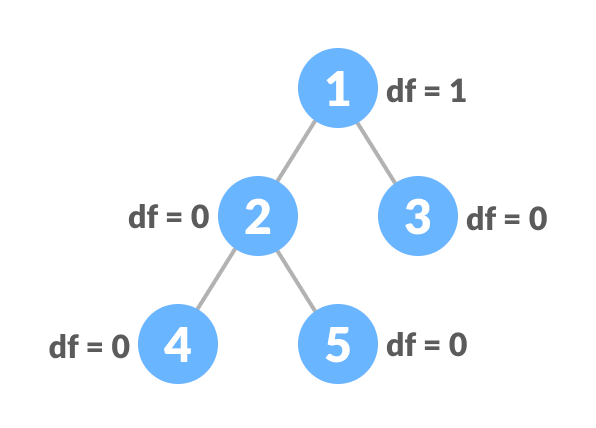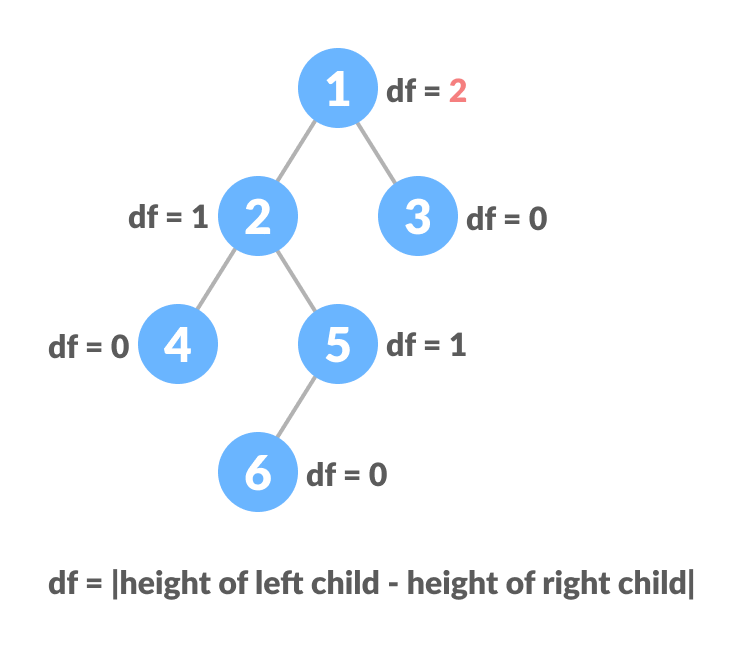# Balanced Binary Tree

In this tutorial, you will learn about a balanced binary tree and its different types. Also, you will find working examples of a balanced binary tree in C, C++, Java and Python.

A balanced binary tree, also referred to as a height-balanced binary tree, is defined as a binary tree in which the height of the left and right subtree of any node differ by not more than 1.

To learn more about the height of a tree/node, visit Tree Data Structure.Following are the conditions for a height-balanced binary tree:

1. difference between the left and the right subtree for any node is not more than one
2. the left subtree is balanced
3. the right subtree is balancedBalanced Binary Tree with depth at each levelUnbalanced Binary Tree with depth at each level

## Python, Java and C/C++ Examples

The following code is for checking whether a tree is height-balanced.

``````# Checking if a binary tree is CalculateHeight balanced in Python

# CreateNode creation
class CreateNode:

def __init__(self, item):
self.item = item
self.left = self.right = None

# Calculate height
class CalculateHeight:
def __init__(self):
self.CalculateHeight = 0

# Check height balance
def is_height_balanced(root, CalculateHeight):

left_height = CalculateHeight()
right_height = CalculateHeight()

if root is None:
return True

l = is_height_balanced(root.left, left_height)
r = is_height_balanced(root.right, right_height)

CalculateHeight.CalculateHeight = max(
left_height.CalculateHeight, right_height.CalculateHeight) + 1

if abs(left_height.CalculateHeight - right_height.CalculateHeight) <= 1:
return l and r

return False

CalculateHeight = CalculateHeight()

root = CreateNode(1)
root.left = CreateNode(2)
root.right = CreateNode(3)
root.left.left = CreateNode(4)
root.left.right = CreateNode(5)

if is_height_balanced(root, CalculateHeight):
print('The tree is balanced')
else:
print('The tree is not balanced')
``````
``````// Checking if a binary tree is height balanced in Java

// Node creation
class Node {

int data;
Node left, right;

Node(int d) {
data = d;
left = right = null;
}
}

// Calculate height
class Height {
int height = 0;
}

class BinaryTree {

Node root;

// Check height balance
boolean checkHeightBalance(Node root, Height height) {

// Check for emptiness
if (root == null) {
height.height = 0;
return true;
}

Height leftHeighteight = new Height(), rightHeighteight = new Height();
boolean l = checkHeightBalance(root.left, leftHeighteight);
boolean r = checkHeightBalance(root.right, rightHeighteight);
int leftHeight = leftHeighteight.height, rightHeight = rightHeighteight.height;

height.height = (leftHeight > rightHeight ? leftHeight : rightHeight) + 1;

if ((leftHeight - rightHeight >= 2) || (rightHeight - leftHeight >= 2))
return false;

else
return l && r;
}

public static void main(String args[]) {
Height height = new Height();

BinaryTree tree = new BinaryTree();
tree.root = new Node(1);
tree.root.left = new Node(2);
tree.root.right = new Node(3);
tree.root.left.left = new Node(4);
tree.root.left.right = new Node(5);

if (tree.checkHeightBalance(tree.root, height))
System.out.println("The tree is balanced");
else
System.out.println("The tree is not balanced");
}
}``````
``````// Checking if a binary tree is height balanced in C

#include <stdio.h>
#include <stdlib.h>
#define bool int

// Node creation
struct node {
int item;
struct node *left;
struct node *right;
};

// Create a new node
struct node *newNode(int item) {
struct node *node = (struct node *)malloc(sizeof(struct node));
node->item = item;
node->left = NULL;
node->right = NULL;

return (node);
}

// Check for height balance
bool checkHeightBalance(struct node *root, int *height) {
// Check for emptiness
int leftHeight = 0, rightHeight = 0;
int l = 0, r = 0;

if (root == NULL) {
*height = 0;
return 1;
}

l = checkHeightBalance(root->left, &leftHeight);
r = checkHeightBalance(root->right, &rightHeight);

*height = (leftHeight > rightHeight ? leftHeight : rightHeight) + 1;

if ((leftHeight - rightHeight >= 2) || (rightHeight - leftHeight >= 2))
return 0;

else
return l && r;
}

int main() {
int height = 0;

struct node *root = newNode(1);
root->left = newNode(2);
root->right = newNode(3);
root->left->left = newNode(4);
root->left->right = newNode(5);

if (checkHeightBalance(root, &height))
printf("The tree is balanced");
else
printf("The tree is not balanced");
}``````
``````// Checking if a binary tree is height balanced in C++

#include
using namespace std;

#define bool int

class node {
public:
int item;
node *left;
node *right;
};

// Create anew node
node *newNode(int item) {
node *Node = new node();
Node->item = item;
Node->left = NULL;
Node->right = NULL;

return (Node);
}

// Check height balance
bool checkHeightBalance(node *root, int *height) {
// Check for emptiness
int leftHeight = 0, rightHeight = 0;

int l = 0, r = 0;

if (root == NULL) {
*height = 0;
return 1;
}

l = checkHeightBalance(root->left, &leftHeight);
r = checkHeightBalance(root->right, &rightHeight);

*height = (leftHeight > rightHeight ? leftHeight : rightHeight) + 1;

if (std::abs(leftHeight - rightHeight >= 2))
return 0;

else
return l && r;
}

int main() {
int height = 0;

node *root = newNode(1);
root->left = newNode(2);
root->right = newNode(3);
root->left->left = newNode(4);
root->left->right = newNode(5);

if (checkHeightBalance(root, &height))
cout << "The tree is balanced";
else
cout << "The tree is not balanced";
}``````